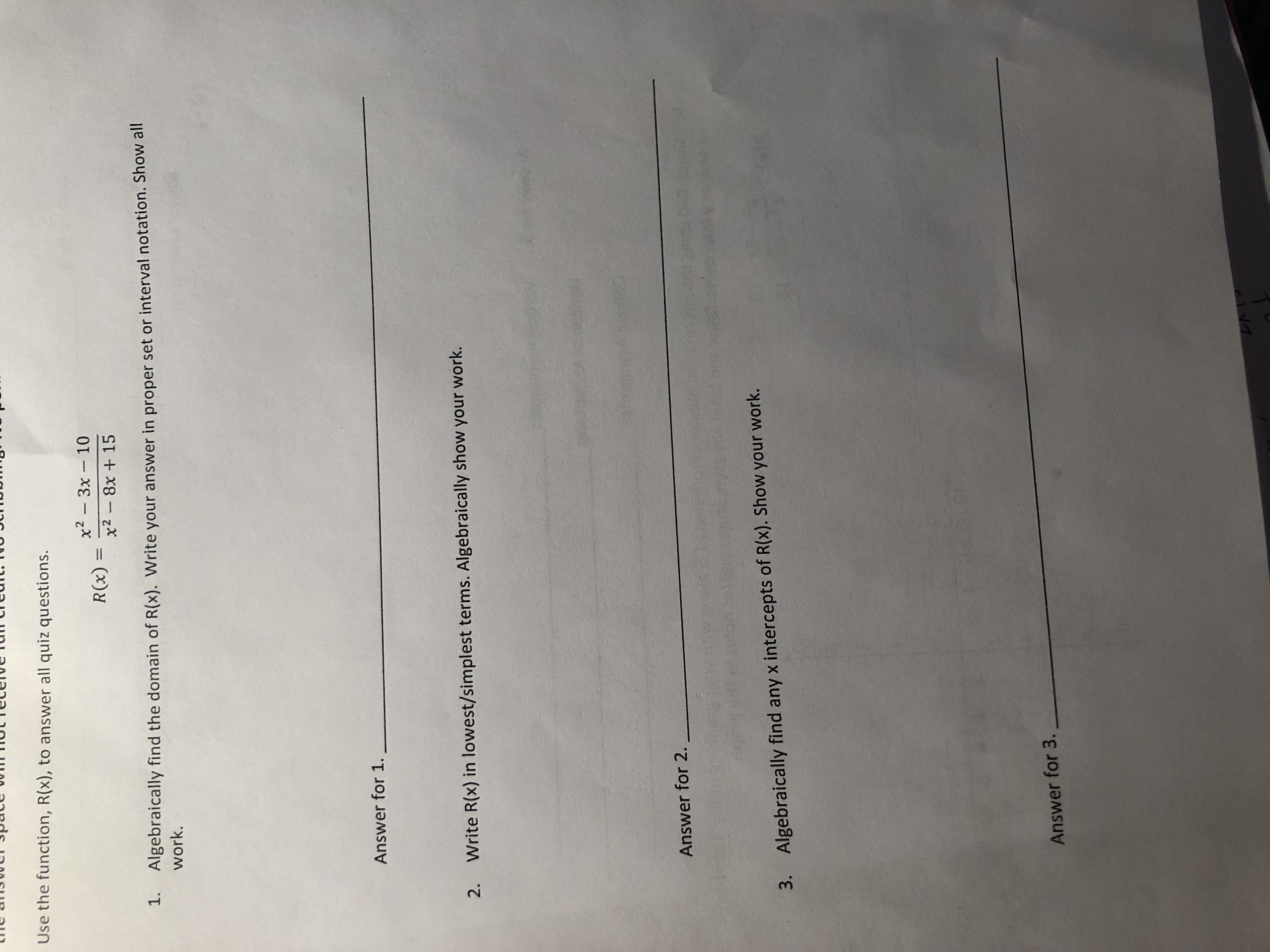# Use the function, R(x), to answer all quiz questions.x2 3x 10x2 8x + 15R(x)1.Algebraically find twork.he domain of R(x). Write your answer in proper set or interval notation. Show allAnswer for 1.,2.Write R(x) in lowest/simplest terms. Algebraically show your work.Answer for 2.3.Algebraically find any x intercepts of R(x). Show your work.Answer for 3.

Question
9 views

Can you help me to solve from 1 to 3. Thank you so much.help_outlineImage TranscriptioncloseUse the function, R(x), to answer all quiz questions. x2 3x 10 x2 8x + 15 R(x) 1. Algebraically find t work. he domain of R(x). Write your answer in proper set or interval notation. Show all Answer for 1., 2. Write R(x) in lowest/simplest terms. Algebraically show your work. Answer for 2. 3. Algebraically find any x intercepts of R(x). Show your work. Answer for 3. fullscreen
check_circle

Step 1

(1) The function is

Step 2

The domain of R(x) can be computed by considering

Step 3

Compute for x....

### Want to see the full answer?

See Solution

#### Want to see this answer and more?

Solutions are written by subject experts who are available 24/7. Questions are typically answered within 1 hour.*

See Solution
*Response times may vary by subject and question.
Tagged in

### Equations and In-equations